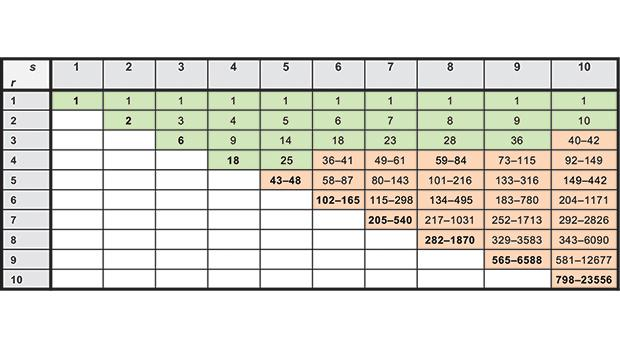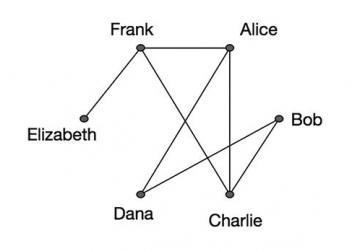Finding order in disorder
Advert

# Finding order in disorderImage 1: Known values of R(r,s) for r and s ranging from 1 to 10. R(s,r) is equal to R(r,s), so the table is symmetric across the diagonal. Data source: https://en.wikipedia.org/wiki/Ramsey%27s_theorem

Ramsey Theory, named after the Cambridge mathematician, philo­sopher and economist Frank P. Ramsey (1903-1930), is a popular area of combinatorial mathematics that can be described as finding order in disorder.

A basic result in this area is that in any group of six people or more, there are three people who know each other or three mutual strangers, that is, the group has a 3-clique (a clique of size 3) or a 3-coclique. Notice that despite the disorder arising from knowing nothing about the group except that its size is at least six, we can always find the desired order, a clique or a coclique of size at least three.

Indeed, let A, B, C, D, E and F be any six people. Suppose A knows at least three people, say B, C and D. If B, C and D are mutual strangers, then we are done; otherwise, two of them know each other and together with A form a 3-clique. Now suppose A knows at most two people. Then, there are at least three people that A does not know, say B, C and D. If B, C and D know each other, then we are done; otherwise, two of them are mutual strangers and together with A form a 3-coclique.

Despite the disorder arising from knowing ‘nothing’, we can always find the desired order

The desired order is not guaranteed if the group consists of 5 people or less. One can check that if A knows E and B only, B knows A and C only, C knows B and D only, D knows C and E only, and E knows D and A only, then among A, B, C, D and E there is neither a 3-clique nor a 3-coclique. Thus, six is the smallest group size that guarantees a 3-clique or a 3-coclique.

A system involving relations is mathematically represented by a graph G. Here G is not the usual plot but simply a set of objects called vertices together with a set of pairs called edges; XY is an edge of G if, and only if, the two objects X and Y are related (for example, know each other; see Image 2). Thus, a graph can model anything from a network (social, neural, computer, transport, communication, etc.) to a molecular structure. The study of graphs is a vast branch of mathematics called graph theory.Image 2: A drawing of a graph representing acquaintances. Source: https://brilliant.org/wiki/wiki-collaboration-graph

The Ramsey number R(r,s) is the smallest number n such that every graph on n objects has an r-clique (r objects, every two of which are related) or an s-coclique (s objects, no two of which are related). Above, we showed that R(3,3) = 6. Motivated by a problem in formal logic, Ramsey showed that R(r,s) exists for any r and s (F.P. Ramsey, On a problem of formal logic, Proceedings of the London Mathematical Society 30 (1930), 264-286). Ramsey Theory originated from this. Image 1 provides known values of R(r,s) for r and s ranging from one to 10. It demonstrates how vast the space for further research is even for determining these values!

Of particular interest are the values R(r,r), called diagonal Ramsey numbers. It is known that R(4,4) = 18. The prolific mathematician Paul Erdős (1913-1996) famously said that if aliens threaten to obliterate the earth unless we determine R(5,5), then we should marshal the world’s best mathematicians and computers, and attempt to find the value, but if they ask for R(6,6), then we would need to launch a preemptive attack!

Peter Borg is an associate professor at the Department of Mathematics within the Faculty of Science of the University of Malta (http://staff.um.edu.mt/peter.borg).

## Did you know?

• The number of ways of arranging a pack of cards is 80,658,175,170,943,878,571,660, 636,856, 403,766,975,289,505,440,883,277,824,000,000,000,00. Thus, if a pack is shuffled properly, the chances are that the order of the cards never occurred before in the history of the universe.

• For a natural number n, we write n! to denote the product 1 x 2 x 3 x ... x n. 10! seconds are six weeks.

• 7 is the only member of the set of numbers from 1 to 10 that never gives another member when multiplied or divided by a member.

• 100 = 123 - 45 - 67 + 89 = 123 + 4 - 5 + 67 - 89 = 123 - 4 - 5 - 6 - 7 + 8 - 9 = 1 + 23 - 4 + 5 + 6 + 78 - 9.

For more trivia, see: www.um.edu.mt/think

## Sound bites

• International scene: David Conlon is a Professor of Discrete Mathematics at University of Oxford. While still a PhD student at University of Cambridge (his adviser was the Fields Medal winner Timothy Gowers), Conlon obtained the best known upper bound on the diagonal Ramsey numbers R(r,r). His paper was published in Annals of Mathematics, one of the top mathematics journals, in 2009 (A new upper bound for diagonal Ramsey numbers, Annals of Mathematics 170 (2009), 941-960). Consequently, he won the European Prize in Combinatorics in 2011.

• Local scene: The degree of a vertex v of a graph is the number of vertices it is related to. In a 2017 paper (Reducing the maximum degree of a graph by deleting vertices, The Australasian Journal of Combinatorics 69(1) (2017), 29-40), Prof. Peter Borg (University of Malta) and his PhD student Kurt Fenech obtained an upper bound on the smallest number of vertices that can be removed from a graph so that the resulting graph has a smaller maximum degree. In a 2018 paper (Reducing the maximum degree of a graph by deleting vertices: the extremal cases, Theory and Applications of Graphs 5(2) (2018), article 5), they determined the graphs that attain the bound. This paper is currently listed as most popular in the journal it is published in.

For more soundbites listen to Radio Mocha on Radju Malta every Monday at 7pm, with a repeat on Thursday at 4pm on Radju Malta 2.

https://www.facebook.com/RadioMochaMalta/

Comments not loading? We recommend using Google Chrome or Mozilla Firefox with javascript turned on.
Comments powered by Disqus
Advert

## Popular Stories## Careers• ### Deployment & Environment Management• ### Finance and Administration Officer...• ### Account Manager - GermanAdvert